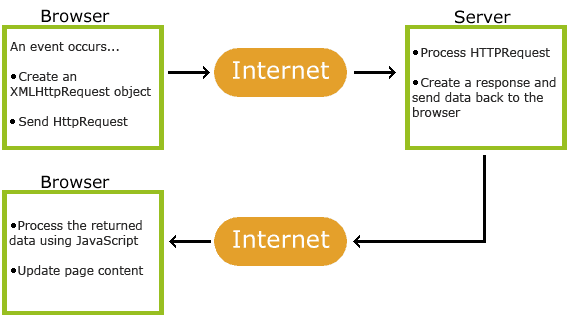# ASP AJAX

## What is AJAX?

AJAX = Asynchronous JavaScript and XML.

AJAX is a technique for creating fast and dynamic web pages.

AJAX allows web pages to be updated asynchronously by exchanging small amounts of data with the server behind the scenes. This means that it is possible to update parts of a web page, without reloading the whole page.

Classic web pages, (which do not use AJAX) must reload the entire page if the content should change.

## How AJAX Works## AJAX is Based on Internet Standards

AJAX is based on internet standards, and uses a combination of:

• XMLHttpRequest object (to exchange data asynchronously with a server)
• JavaScript/DOM (to display/interact with the information)
• CSS (to style the data)
• XML (often used as the format for transferring data)

AJAX applications are browser- and platform-independent!

Google Suggest is using AJAX to create a very dynamic web interface: When you start typing in Google's search box, a JavaScript sends the letters off to a server and the server returns a list of suggestions.

## Start Using AJAX Today

In our ASP tutorial, we will demonstrate how AJAX can update parts of a web page, without reloading the whole page. The server script will be written in ASP.

## AJAX ASP Example

The following example will demonstrate how a web page can communicate with a web server while a user type characters in an input field:

### Example

Start typing a name in the input field below:

First name:

Suggestions:

## Example Explained

In the example above, when a user types a character in the input field, a function called "showHint()" is executed.

The function is triggered by the onkeyup event.

Here is the HTML code:

### Example

<html>
<script>
function showHint(str) {
if (str.length == 0) {
document.getElementById("txtHint").innerHTML = "";
return;
} else {
var xmlhttp = new XMLHttpRequest();
if (this.readyState == 4 && this.status == 200) {
document.getElementById("txtHint").innerHTML = this.responseText;
}
};
xmlhttp.open("GET", "gethint.asp?q=" + str, true);
xmlhttp.send();
}
}
</script>
<body>

<p><b>Start typing a name in the input field below:</b></p>
<form>
First name: <input type="text" onkeyup="showHint(this.value)">
</form>
<p>Suggestions: <span id="txtHint"></span></p>
</body>
</html>
Try it Yourself »

Code explanation:

First, check if the input field is empty (str.length == 0). If it is, clear the content of the txtHint placeholder and exit the function.

However, if the input field is not empty, do the following:

• Create an XMLHttpRequest object
• Create the function to be executed when the server response is ready
• Send the request off to an ASP file (gethint.asp) on the server
• Notice that q parameter is added gethint.asp?q="+str
• The str variable holds the content of the input field

## The ASP File - "gethint.asp"

The ASP file checks an array of names, and returns the corresponding name(s) to the browser:

<%
response.expires=-1
dim a(30)
'Fill up array with names
a(1)="Anna"
a(2)="Brittany"
a(3)="Cinderella"
a(4)="Diana"
a(5)="Eva"
a(6)="Fiona"
a(7)="Gunda"
a(8)="Hege"
a(9)="Inga"
a(10)="Johanna"
a(11)="Kitty"
a(12)="Linda"
a(13)="Nina"
a(14)="Ophelia"
a(15)="Petunia"
a(16)="Amanda"
a(17)="Raquel"
a(18)="Cindy"
a(19)="Doris"
a(20)="Eve"
a(21)="Evita"
a(22)="Sunniva"
a(23)="Tove"
a(24)="Unni"
a(25)="Violet"
a(26)="Liza"
a(27)="Elizabeth"
a(28)="Ellen"
a(29)="Wenche"
a(30)="Vicky"

'get the q parameter from URL
q=ucase(request.querystring("q"))

'lookup all hints from array if length of q>0
if len(q)>0 then
hint=""
for i=1 to 30
if q=ucase(mid(a(i),1,len(q))) then
if hint="" then
hint=a(i)
else
hint=hint & " , " & a(i)
end if
end if
next
end if

'Output "no suggestion" if no hint were found
'or output the correct values
if hint="" then
response.write("no suggestion")
else
response.write(hint)
end if
%>

AJAX can be used for interactive communication with a database.

## AJAX Database Example

The following example will demonstrate how a web page can fetch information from a database with AJAX:

### Example

Customer info will be listed here...

## Example Explained - The HTML Page

When a user selects a customer in the dropdown list above, a function called "showCustomer()" is executed. The function is triggered by the "onchange" event:

<!DOCTYPE html>
<html>
<script>
function showCustomer(str)
{
if (str=="")
{
document.getElementById("txtHint").innerHTML="";
return;
}
if (window.XMLHttpRequest)
{// code for IE7+, Firefox, Chrome, Opera, Safari
xmlhttp=new XMLHttpRequest();
}
else
{// code for IE6, IE5
xmlhttp=new ActiveXObject("Microsoft.XMLHTTP");
}
{
{
document.getElementById("txtHint").innerHTML=this.responseText;
}
}
xmlhttp.open("GET","getcustomer.asp?q="+str,true);
xmlhttp.send();
}
</script>
<body>

<form>
<select name="customers" onchange="showCustomer(this.value)">
<option value="">Select a customer:</option>
<option value="ALFKI">Alfreds Futterkiste</option>
<option value="NORTS ">North/South</option>
<option value="WOLZA">Wolski Zajazd</option>
</select>
</form>
<

<div id="txtHint">Customer info will be listed here...</div>

</body>
</html>

Source code explanation:

If no customer is selected (str.length==0), the function clears the content of the txtHint placeholder and exits the function.

If a customer is selected, the showCustomer() function executes the following:

• Create an XMLHttpRequest object
• Create the function to be executed when the server response is ready
• Send the request off to a file on the server
• Notice that a parameter (q) is added to the URL (with the content of the dropdown list)

## The ASP File

The page on the server called by the JavaScript above is an ASP file called "getcustomer.asp".

The source code in "getcustomer.asp" runs a query against a database, and returns the result in an HTML table:

<%
response.expires=-1
sql="SELECT * FROM CUSTOMERS WHERE CUSTOMERID="
sql=sql & "'" & request.querystring("q") & "'"

conn.Provider="Microsoft.Jet.OLEDB.4.0"
conn.Open(Server.Mappath("/datafolder/northwind.mdb"))